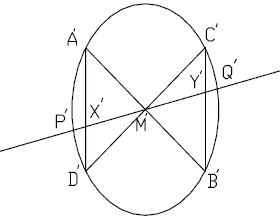# Butterfly theorem, a Projective Proof

The Butterfly Theorem is an obvious corollary of the following statement. This observation and the proof that follows are due to Mikhail Goldenberg (The Ingenuity Project, Baltimore, MD) and Mark Kaplan (Towson State University)

### Theorem

Let L be a nondegenerated second degree curve. Suppose, three lines in the plane of L concur at the point M. Let points A, B; P, Q; and C, D be the intersections of these lines with L, and let AD and BC intersect PQ at X and Y, respectively. Finally, let N be the point harmonic conjugate to M with respect to the pair of points (P, Q). Then N is harmonic conjugate to M with respect to the pair of points (X, Y). In short,

(P, Q; M, N) = -1 ⇒ (X, Y; M, N) = -1.### Proof

First, note that if a rectangle can be inscribed in a nondegenerated second degree curve, then the curve is a central one and its center coincides with the center of the rectangle. Second, the projective transformation preserves the cross ratio. Therefore, it is sufficient to prove the theorem for some projective image of the given configuration.

There exists a projective transformation of the plane of L which maps the quadrilateral ACBD onto a square A'C'B'D'. The image M' of the point M is the center of the square which is, as observed, the center of the image L' of the curve L. Therefore, M' is the midpoint of both segments, P'Q' and X'Y' , and the point N' is at infinity on the line P'Q' because (P, Q; M, N) = -1. So, N' is the harmonic conjugate point for M' with respect to (X', Y').This completes the proof.### Butterfly Theorem and Variants